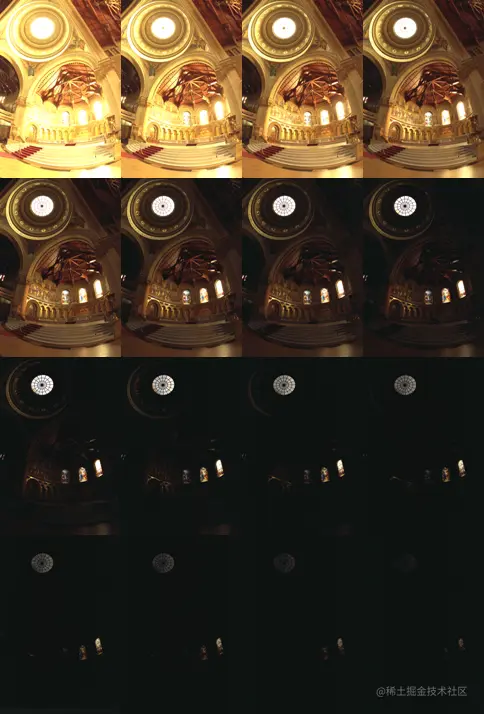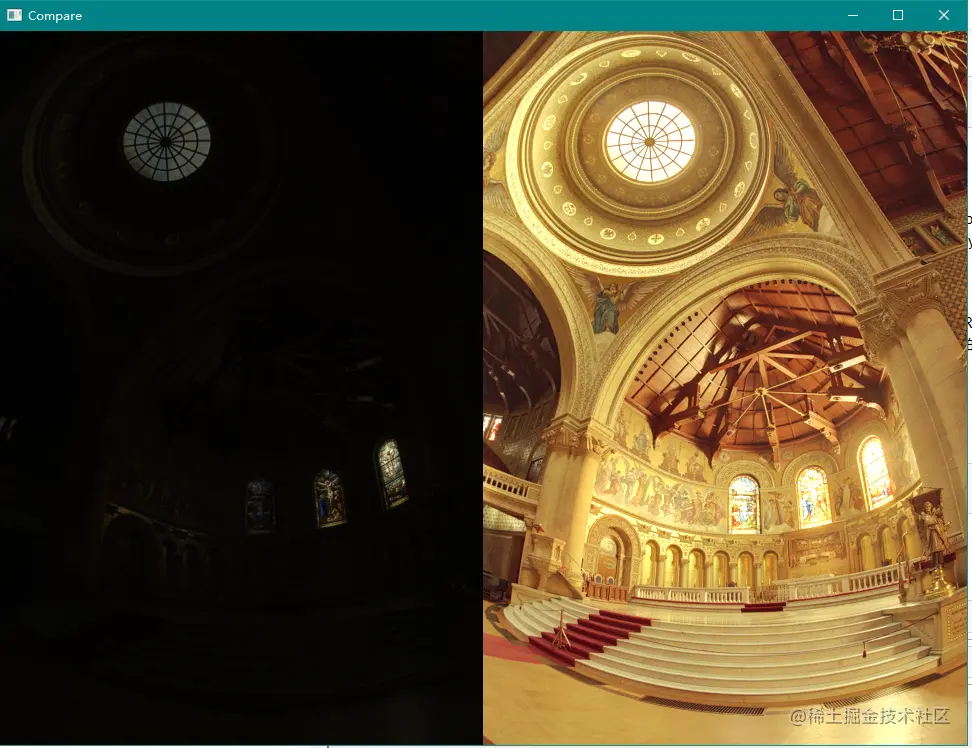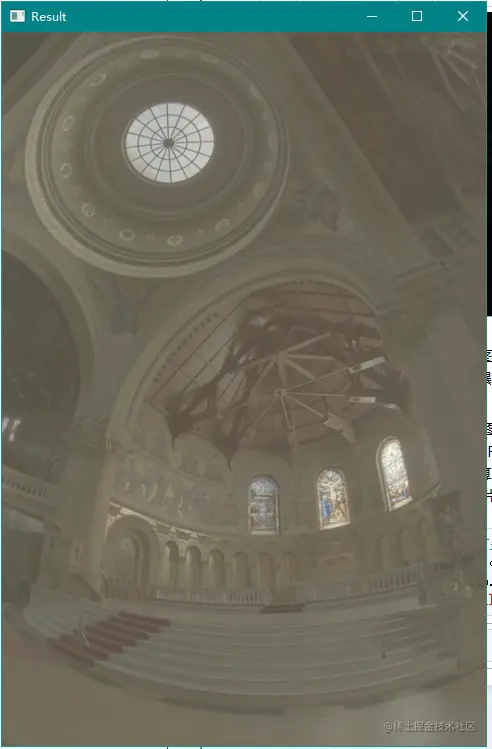﻿ Python中OpenCV Tutorials 20  高动态范围成像的实现步骤_python_脚本之家
python# Python中OpenCV Tutorials 20  高动态范围成像的实现步骤

## 二、曝光序列## 三、代码演示

```from __future__ import print_function
from __future__ import division
import cv2 as cv
import numpy as np
import argparse
import os```
```def cv_show(name, img):
cv.imshow(name, img)
cv.waitKey(0)
cv.destroyAllWindows()```
```def compare(imgs):
#  for i in range(len(imgs)):
#       imgs[i][:,-3:-1,:] = [255,255,255]
res = np.hstack(imgs)
cv_show('Compare', res)```
```def loadExposureSeq(path):
images = []
times = []
with open(os.path.join(path, 'list.txt')) as f:
for line in content:
tokens = line.split()
# 便于之后的逆CRF操作
times.append(1 / float(tokens))
return images, np.asarray(times, dtype=np.float32)```
```# jupyter 难以手动输入参数，故使用绝对路径
#parser = argparse.ArgumentParser(description='Code for High Dynamic Range Imaging tutorial.')
# parser.add_argument('--input', type=str, help='Path to the directory that contains images and exposure times.')
# args = parser.parse_args()
# if not args.input:
#     parser.print_help()
#     exit(0)
# images, times = loadExposureSeq(args.input)
images, times = loadExposureSeq('exposures/')
calibrate = cv.createCalibrateDebevec()
response = calibrate.process(images, times)
merge_debevec = cv.createMergeDebevec()
hdr = merge_debevec.process(images, times, response)
tonemap = cv.createTonemap(2.2)
ldr = tonemap.process(hdr)
merge_mertens = cv.createMergeMertens()
fusion = merge_mertens.process(images)```
```cv.imwrite('fusion.png', fusion * 255)
cv.imwrite('ldr.png', ldr * 255)
cv.imwrite('hdr.hdr', hdr)```
`True`

## 四、解释

### 1. 加载图像和曝光时间

`images, times = loadExposureSeq('exposures/')`
```# 查看数据集中曝光图像个数
len(images)```
`16`

memorial00.png 0.03125

memorial01.png 0.0625

...

memorial15.png 1024

### 2. 估计相机响应

```calibrate = cv.createCalibrateDebevec()
response = calibrate.process(images, times)```
• 用法如下：

cv.createCalibrateDebevec( [, samples[, lambda_[, random]]] ) -> retval

• 参数含义：
• samples ：number of pixel locations to use
• lambda ：smoothness term weight. Greater values produce smoother results, but can alter the response.
• random ：if true sample pixel locations are chosen at random, otherwise they form a rectangular grid.

### 3. 形成HDR图像

```merge_debevec = cv.createMergeDebevec()
# 利用逆CRF形成HDR图像
hdr = merge_debevec.process(images, times, response)```
• 用法如下：

cv.createMergeMertens( [, contrast_weight[, saturation_weight[, exposure_weight]]] ) -> retval

• 参数含义：
• contrast_weight ：contrast measure weight. See MergeMertens.
• saturation_weight： saturation measure weight
• exposure_weight ：well-exposedness measure weight

### 4. 对 HDR 图像进行色调映射

```tonemap = cv.createTonemap(2.2)
ldr = tonemap.process(hdr)
cv_show('Result', ldr)```
• 用法如下： cv.createTonemap( [, gamma] ) -> retval
• 参数含义：
• gamma ：positive value for gamma correction. Gamma value of 1.0 implies no correction, gamma equal to 2.2f is suitable for most displays. Generally gamma > 1 brightens the image and gamma < 1 darkens it.

### 5. 实现曝光融合

```merge_mertens = cv.createMergeMertens()
fusion = merge_mertens.process(images)```

`compare([ldr,fusion])````# 修改gamma使整幅图像变亮
tonemap = cv.createTonemap(10)
ldr = tonemap.process(hdr)
cv_show('Result', ldr)```## 五、补充资源

• Paul E Debevec and Jitendra Malik. Recovering high dynamic range radiance maps from photographs. In ACM SIGGRAPH 2008 classes, page 31. ACM, 2008. 
• Mark A Robertson, Sean Borman, and Robert L Stevenson. Dynamic range improvement through multiple exposures. In Image Processing, 1999. ICIP 99. Proceedings. 1999 International Conference on, volume 3, pages 159–163. IEEE, 1999. 
• Tom Mertens, Jan Kautz, and Frank Van Reeth. Exposure fusion. In Computer Graphics and Applications, 2007. PG'07. 15th Pacific Conference on, pages 382–390. IEEE, 2007. -range_imaging
• Recovering High Dynamic Range Radiance Maps from Photographs (webpage) www.pauldebevec.com/Research/HD…